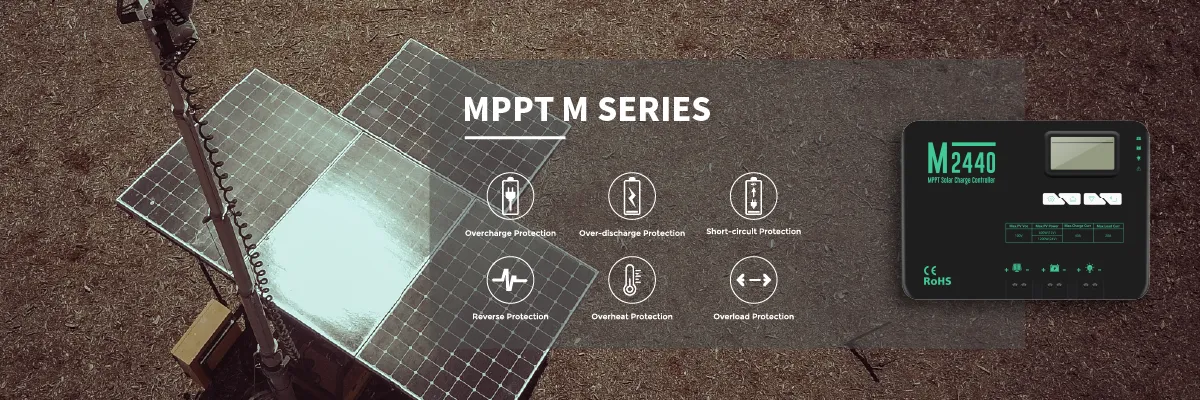8888888888888888888888888888888888888888888888888888888888888888888888888888888888888888888888888888888888888888888888888888888888888888888888888888888888888888888888888888888888888888888888888888888888888888888888888888888888888888888888888888888888888888888888888888888888888888888888888888888888888888888888888888888888888888888888888888888888888888888888888888888888888888888888888888888888888888888888888888888888888888888888888888888888888888888888888888888888888888888888888888888888888888888888888888888888888888888888888888888888888888888888888888888888888888

# How to Choose the Right Solar Charge Controller

2023.09.06There are many different kinds of solar charge controllers, but the most common are controllers for 12, 24, and 48-volt systems. The amperage rating typically ranges from 1 amp to 80 amps, and the voltage ranges from 6 to 600 volts.

If you use two parallel strings of modules in your 48-volt system, you can produce 16.1 amps at 48 volts if one module produces 8.05 amps. It is quite common for current levels to increase due to light reflections or clouds at irregular intervals. Therefore, we increased the charge controller amperage by 25%, bringing it down to 20.13 amps at the minimum. After migrating to our catalog, we found a 30a mppt solar charge controller that matches very closely. It would not be a problem to upgrade to a larger controller, other than the cost. It would also allow you to upgrade your system later on down the road if your load demands change or if you find you need more power.

It was once assumed that your solar panel array and battery would have the same nominal voltage, and you would also select the same voltage for your charge controller. Due to the widespread availability of more efficient charging technology, known as Maximum Power Point Tracking (MPPT), many charge controller models are now compatible with this school of thought. By design, MPPT charge controllers convert the higher voltage of the solar panel array down to the lower voltage of your battery bank. This is one of the key benefits of this technology.

The MPPT Charge Controller also helps you save some money on wiring costs. You can use smaller gauge wiring into the charge controller when you have a solar module array with high voltage. As solar modules can be up to 100 feet away from a charge controller (or more!) it is usually an important target for the project to keep wiring costs to a minimum. If you double the voltage (e.g., from 12 to 24 or 48 volts), the current flowing through the wires will be reduced by half every time, so you will use a lot less copper.

Example of Sizing an MPPT Charge Controller

As an example, your solar panel array could be 3,000 watts with a battery bank of 48 volts DC and operate at 93.3 volts DC. MPPT charge controllers are rated based on the amount of output current they can handle, not the amount of input current they can handle from solar panels. In order to calculate the output current that the charge controller will have to handle, we use the following formula:

Power = Volts x Amps
Here we know the power is 3,000 Watts, the battery bank is 48 volts, so:
3,000 Watts = 48 volts x Amps
which gives us:
Amps = 3,000 Watts/ 48 volts
Amps = 62.5A

To account for any special conditions that might cause a solar module array to produce more power than it is normally rated for (such as sunlight reflecting off snow, water, exceptionally bright conditions, etc.), we still want to adjust this value by 25%. 62.5A multiplied by 25% is now 78.13A. We would probably choose an 80 Amp MPPT Charge Controller, like Outback Power's FlexMax 80, in this case.

Another Benefit of MPPT Charge Controllers

With MPPT charge controllers, you can also use them with solar modules whose voltages do not match your typical system voltage (e.g. 12, 24 or 48V) since they can handle a higher input voltage from the solar module array than the battery bank's voltage. With an MPPT charge controller, you can efficiently charge a solar module with a nominal voltage of 31.1 volts and a battery bank with a voltage of 48 volts.

You should keep in mind that MPPT charge controllers have a maximum system voltage they can handle from solar modules. In order to prevent harm to the controller, you need to make sure that the solar module array voltage does not exceed this limit. If the open circuit voltage of the solar module array exceeds this value, you should take steps to correct it. A 25% margin of error will be sufficient to cover the possibility that an array's voltage will actually increase as it gets colder. If you give yourself a 20% margin of error, you will be fine.

Here's an example:

For a nominal voltage of 93.3 volts, we will use 12 SolarWorld 250 Watt solar modules with four parallel strings of three in series and a 48-volt battery bank. Schneider Conext MPPT 60 150 will be used as a charge controller. We find on the module's specification page that each module has an open circuit voltage of 37.8V. The array has three times that voltage because there are three modules in series. Therefore, the array's open circuit voltage is 113.4V x 3 = 37.8V. Using a safety factor of 25%, we get 141.75V. The Conext MPPT 60 150 has a maximum operating voltage of 150 volts, so you're good to go!

Top
Scan the QR code Close